Ch 4. Fundamental Laws (Integral Anal.) Multimedia Engineering Fluids ConservationMass LinearMomentum Moment ofMomentum ConservationEnergy
 Chapter 1. Basics 2. Fluid Statics 3. Kinematics 4. Laws (Integral) 5. Laws (Diff.) 6. Modeling/Similitude 7. Inviscid 8. Viscous 9. External Flow 10. Open-Channel Appendix Basic Math Units Basic Equations Water/Air Tables Sections Search eBooks Dynamics Fluids Math Mechanics Statics Thermodynamics Author(s): Chean Chin Ngo Kurt Gramoll ©Kurt GramollFLUID MECHANICS - CASE STUDY SOLUTION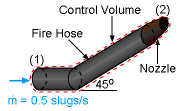Problem Description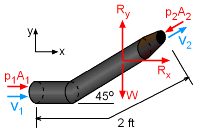Forces on the Control Volume First select a control volume to include the hose, nozzle and water inside the hose, as shown by the red dashed lines in the figure. Rx and Ry represent the reaction force components acting on the hose. W is the weight of the water inside the hose. The linear momentum equation for steady flow is given by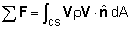which can be written in terms of x- and y- component as follows:     x-component:    p1A1 - p2A2cos45o + Rx                       = V1ρ(-V1)A1 + V2cos45oρ(V2)A2     y-component:    - p2A2sin45o - W + Ry = V2sin45oρ(V2)A2 The gage pressure at the nozzle outlet is zero (i.e., p2 = 0) since water is discharged to the atmosphere. According to the principle of conservation of mass,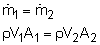The linear momentum equations can be simplified to yield the reaction force in the x- direction: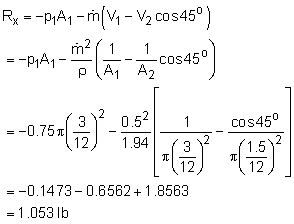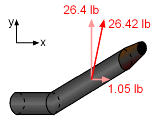Required Resultant Force for Holding the Hose in Place and the reaction force in the y-direction: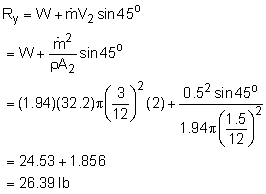Hence, the resultant force required to hold the hose in place is given by F = 1.05i + 26.4j lb.

Practice Homework and Test problems now available in the 'Eng Fluids' mobile app
Includes over 250 problems with complete detailed solutions.
Available now at the Google Play Store and Apple App Store.